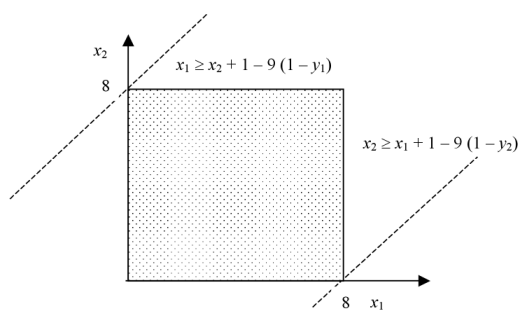# BIGM Relaxation for a linear disjunction

Suppose the following disjunction with i terms: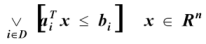(1)

The "Big-M" relaxation for the previous disjunction can be defined as follows: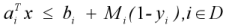(2)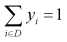(3)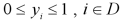(4)

Where Mi is a parameter whose value is large enough such that if yi = 0, then (2) becomes redundant. If yi = 1 then constraint aiTx <= bi must be satisfied. The tightest value for Mi is given by: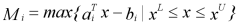Example:
As an illustration, consider the following disjunction: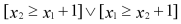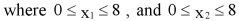(5)

The feasible region for the disjunction is: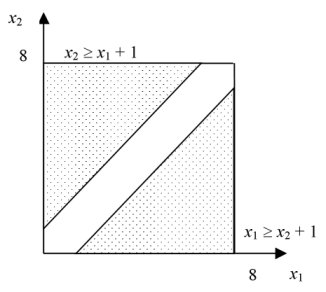The Big-M relaxation of (5) is given by: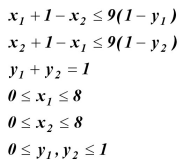(BM1)

The feasible region of the BigM relaxation is: